# How to convert text strings that represent numbers to numbers

Feb 04, 2020 • edited Feb 05, 2020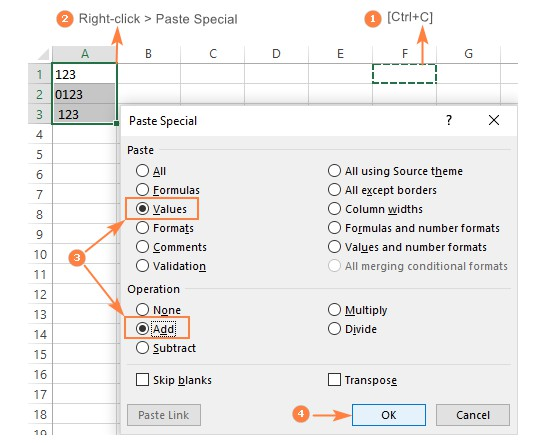Sometimes values in your Excel worksheets look like numbers, but they don't add up, don't multiply and produce errors in formulas. A common reason for this is numbers formatted as text. In many cases Microsoft Excel is smart enough to convert numerical strings imported from other programs to numbers automatically. But sometimes numbers are left formatted as text causing multiple issues in your spreadsheets. This tutorial will teach you how to convert strings to “true” numbers.

It’s common to find numbers stored as text in Excel. This leads to incorrect calculations when you use these cells in Excel functions such as SUM and AVERAGE (as these functions ignore cells that have text values in it). In such cases, you need to convert cells that contain numbers as text back to numbers.

How to Convert Text to Numbers in Excel - learn how to correct this very common Excel issue with 5 different methods. Such issues usually occur when you copy numbers from another type of document (Word or Notepad etc.)

By default, text is left-aligned and numbers are right-aligned. This example teaches you how to convert ‘text strings that represent numbers’ to numbers.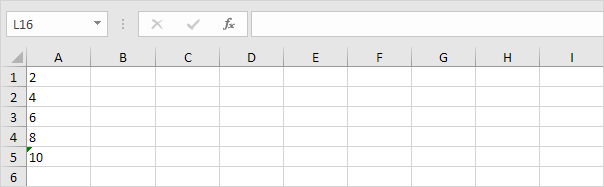1. Select the range A1:A4 and change the number format to General.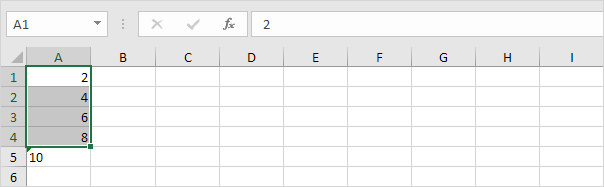2. Numbers preceded by an apostrophe are also treated as text. Select cell A5 and manually remove the apostrophe.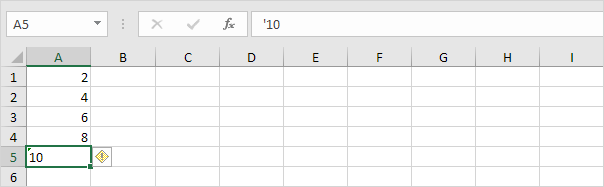3a. You can also combine step 1 and 2 by adding an empty cell to the range A1:A5. By doing this, you let Excel know that these text strings are numbers. Copy an empty cell.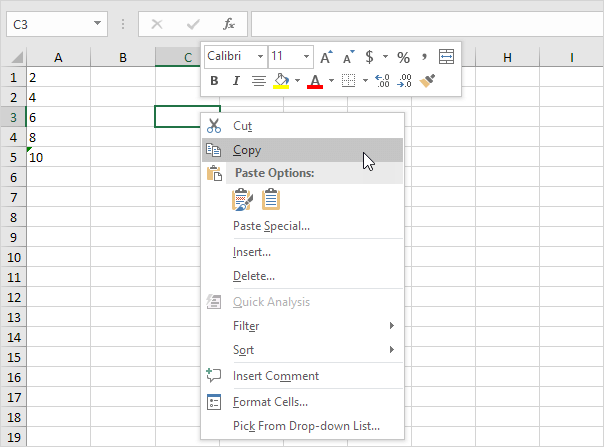3b. Select the range A1:A5, right click, and then click Paste Special.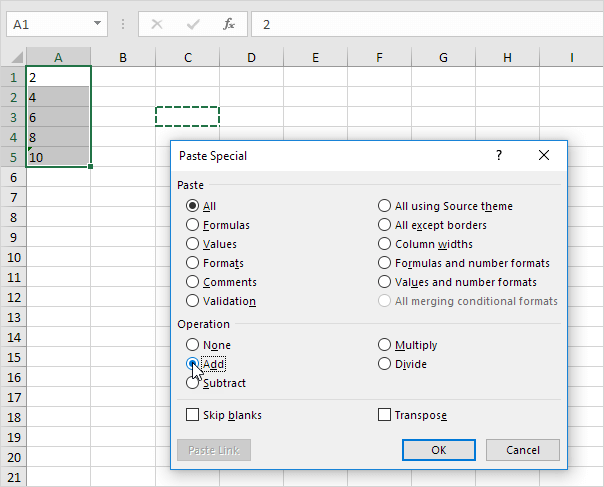3d. Click OK.

Result. All numbers are right-aligned and treated as numbers.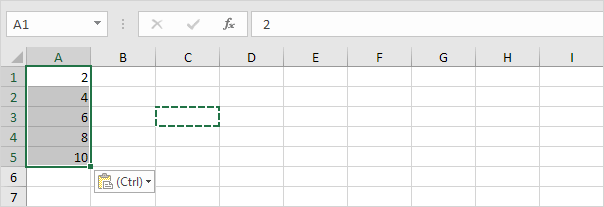4a. You can also use the VALUE function.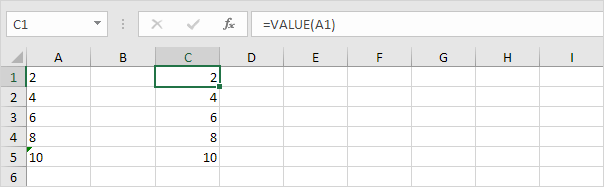4b. Here's another example. Use the RIGHT function (or any other text function) to extract characters from a text string and then use the VALUE function to convert these characters to a number.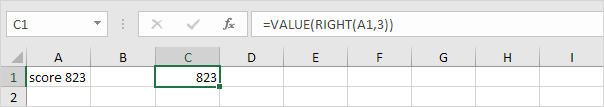#How To#Tutorial#Format Cells#Text to Numbers

How to convert numbers to text in excel

The Currency and Accounting format in Excel